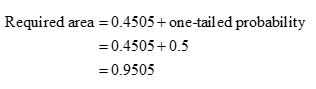# The area under the standard normal curve from zero to z is 0.4505 and z is positive. The value of z is

Question
1 views

The area under the standard normal curve from zero to z is 0.4505 and z is positive. The value of z is

check_circle

Step 1

Normal distribution is a symmetrical distribution so the area is equally distributed on both tails of the distribution.Step 2

The positive z value is obtained below:

Procedure for finding the z-value is listed below:

1. From the table of standard normal distribution, locate the probability value of 0.9505.
2. Move left until the first column is reached. Note the value as 1.6
3. Move upward until the top row is reached. Note the value as 0.05.
4. The intersection of the row and column values gives the area to the two tail of...

### Want to see the full answer?

See Solution

#### Want to see this answer and more?

Solutions are written by subject experts who are available 24/7. Questions are typically answered within 1 hour.*

See Solution
*Response times may vary by subject and question.
Tagged in